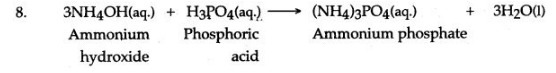ICSESolutions.com provides ICSE Solutions for Class 10 Chemistry Chapter 3 Acids, Bases and Salts for ICSE Board Examinations. We provide step by step Solutions for ICSE Chemistry Class 10 Solutions Pdf. You can download the Class 10 Chemistry ICSE Textbook Solutions with Free PDF download option.

## ICSE Class 10 Chemistry Chapter 3 Acids, Bases and Salts Solutions

### Short Questions

Question 1: Solution A is a strong acid, solution B is a weak acid, solution C is a strong alkali.
(i) Which solution contains solute molecules in addition to water molecules.
(ii) Which solution could be a solution of glacial acetic acid ?
(iii) Give an example of a solution which is a weak alkali.
Answer: (i) B (ii) B (iii) Ammonium hydroxide

Question 2: (i) What is basicity of an acid. Give examples.
(ii) Give the basicity of nitric acid, acetic acid and phosphoric acid.
(iii) State two properties of an acid.
(iv) On what factors the strength of an acid depend?
(v) Name an acid used : (a) as a flavour and to preserve food (b) in a drink (c) to remove ink spots (d) as an eyewash.
Answer: (i) The number of replaceable hydrogen atoms, present in one molecule of acid is known as the basicity of the acid. For example hydrochloric acid is a monobasic acid, sulphuric acid is a dibasic acid and phosphoric acid is a tribasic acid.
(ii) Nitric acid (HNO3) —> Monobasic
(iii) (a) An add turns methyl orange into pink and blue litmus to red.
(b) An add with a base forms salt and water only.
NaOH + HCl —> NaCl + H2O
(alkali) (acid)     (Salt) (water)
(iv) The strength of an acid depends on the following :
(a) Reactivity of the acid. (b) Degree of ionisation to H+ ion.
(v) (a) Citric add. (b) Carbonic acid.
(c) Oxalic add. (d) Boric acid.

Question 3: (i) What is acidity of a base. Give examples.
(ii) State three properties of an alkali.
Answer: (i) The number of hydroxyl ions that one molecule of a base gives on dissociation is known as acidity of a base.
e.g. The acidity of sodium hydroxide (NaOH) is one, it is a monoacid, the acidity of Ca(OH)2 is two, if is a diacid base, Al(OH)3 is a triacid base.
(ii) An alkali turns :
(a) Red litmus blue (b) Phenolphthalein to pink (c) Methyl orange to yellow.

Question 4: Name the kind of particles present in:
(i) Sodium hydroxide solution. (ii) Carbonic acid (iii) Sugar solution.
Answer: (i) Sodium ions and hydroxide ions.
(ii) Carbonic add molecules, carbonate ions and hydronium ions.
(iii) Sugar molecules and water molecules.

Question 5: Solution A is a sodium hydroxide solution. Solution B is a weak acid. Solution C is dilute sulphuric add. Which solution will
(i) liberate sulphur dioxide from sodium sulphite.
(ii) give a white precipitate with zinc sulphate solution.
(iii) contain solute molecules and ions ?
Answer: (i) C (ii) A (iii) B

Question 6: (i) What are indicators?
(ii) Name three indicators and their colours in different mediums.
(iii) What is reaction of neutralization?
(iv) Give two practical application of Neutralization.
Answer: (i) Indicators are complex organic compounds, which are used to distinguish between acidic and alkaline solutions by the abrupt change in their colour, e.g. litmus, methyl orange and phenolphthalein.
(ii) The three indicators and their colours in different medium are given below:

 Indicator Colour in acidic solution Colour in alkaline solution (i) Litmus Red Blue (ii) Methyl orange Red Yellow (iii) Phenolphthalein Colourless Pink

Neutralization is an exothermic reaction, in which an acid reacts with a base to form salt and water only.
NaOH + HNO3 —> NaNO3 + H2O.
Base     Acid             Salt           water
In the reaction of neutralization, the hydrogen ions of an acid combine with the hydroxyl ions of alkali to form water, at the same time salt is formed.
(iv) (a) Acidity of soil is reduced by adding slaked lime.
(b) Antacid tablets are given to a person suffering from acidity.

Question 7: (i) Explain pH value of a solution.
(ii) What is the pH of:
(a) Pure water, (b) Milk, and (c) Human blood.
(iii) The pH value of three solutions is given below. Which one of them is acidic, neutral and alkaline in nature?
(a) pH = 7, (b) pH = 10 and (c) pH = 3.
(iv) What is pH number assigned to a solution?
Answer: (i) pH value of a solution is the negative logarithm to the base 10 of hydrogen ion concentration expressed in gram-ions per litre.

pH = log10[H+]

• pH value = 7 indicates a neutral solution.
• pH value > 7 indicates an alkaline solution.
• pH value < 7 indicates an acidic solution.

(ii) The pH of:
(a) Pure water is 7 (b) Milk is 6.6 (c) Human blood is 7.3
(iii) (a) Neutral, (b) Alkaline and (c) Acidic.
(iv) It is a number assigned to express the acidity or alkalinity of a solution which varies from 1 to 14. A pH number less than 7 indicates acidity, while pH number more than 7 indicates alkalinity. The pH of pure water is 7 and it is neutral.

Question 8: (i) (a) What is the purpose of the pH scale?
(b) What is the pH of pure water.
(c) A is a soluble acidic oxide, B is a soluble base compared to the pH of pure water, what will be the pH of: (A) a solution of A, (B) a solution of B.
(ii) Taking sodium carbonate as an example, give the meaning of the following terms:
(a) Water of crystallization (b) Anhydrous (c) Efflorescence.
Answer: (i) (a) To measure the degree of acidity or alkalinity.
(b) The pH of pure water is equal to 7.
(c) (A) The pH is less than 7. (B) The pH is more than 7.
(ii) (a) Water of crystallization is that a definite number of water molecules which are to a crystal when it is crystallizing out from an aqueous solution, e.g. Sodium carbonate crystal contains 10 molecules of water i.e. Na2CO3. 10H2O.
(b) When Na2CO3. 10H2O is heated, the water of crystallization is evaporated leaving anhydrous sodium carbonate Na2CO3.
(c) The loss of water by a hydrate on exposure to air is called efflorescence, e.g. Na2CO3. 10H2O on exposure to air loses 9 molecules of water of crystallization.
Na2CO3. 10H2O —> Na2CO3. H2O + 9H2O.

Question 9: A solution has a pH of 7 explain how you would :
(i) (a) Increase its pH, (b) Decrease its pH.
(ii) If a solution changes the colour of litmus red to blue what can you say about its pH?
(iii) What can you say about the pH of a solution that liberates carbon dioxide from sodium carbonate.
(i) (a) pH is increased by adding any caustic alkali to the solution.
(b) pH is decreased by adding any mineral acid to the solution.
(ii) pH of solution is more than 7.
(iii) pH of solution is less than 7.

Question 10: (i) The pH value is utilised in medicine and agriculture. Explain.
(ii) The pH value of pure water is 7. Compare the pH values of sulphur dioxide solution and ammonia solution with that of pure water.
(iii) How does pH value of a solution and hydrogen ion concentration of solution vary ?
Answer: (i) (a) In Medicine: The pH values of urine and blood are used to diagnose various diseases, (b) In Agriculture: The pH of soil is checked to ensure fast growth of crops. For example, citrus fruits need slightly alkaline soil, rice crop needs an acidic soil, sugarcane needs a neutral soil.
(ii) Sulphur dioxide is a non-metal oxide, such oxides are acidic with pH less then 7. Ammonia solution is alkaline with pH more than 7.
(iii) Both varies inversely. It is seen-that lower the pH value the greater the H+ ion concentration and higher the pH value the lower the H+ ion concentration.

Question 11: You are provided with the following chemicals :
Ammonium hydroxide, chlorine, copper oxide, iron, lead nitrate and dilute sulphuric acid; using only chemicals of this given list, write equation for the following salt preparations:
(i) A salt by direct combination.
(ii) A soluble salt by neutralisation of an alkali.
(iii) A soluble salt from an insoluble base.
(iv) A salt by double decomposition (Precipitation).
(v) A soluble salt from a metal.Question 12: How many types of salts can be prepared from orthophosphoric acid ? Is there any difference in the salts formed by the acid ?
Answer: There are three types of salts prepared from orthophosphoric acid [H3PO4], two acidic salts and one normal salt. These salts are obtained by the stepwise replacement of hydrogen atom from orthophosphoric acid. For example: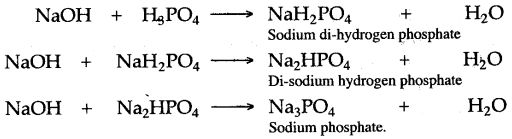The acidic salts obtained from orthophosphoric acid have the capacity to ionize further to yield hydrogen ion and thus they can change blue litmus paper red, whereas normal salt does not.

Question 13: Zinc sulphate is called a “salt”, sulphur dioxide an “acidic oxide” and lead monoxide a “basic oxide”. What is meant by these terms ?
Answer: (i) Zinc sulphate is a salt, because it is formed by the complete replacement of the hydrogen of sulphuric acid by zinc.
Zn + H2SO4 —> ZnSO4 (Zinc sulphate) + H2
(ii) Sulphur dioxide is an acidic oxide, because it is an oxide of non-metal, which dissolves in water to form an acidlcnown as sulphurous acid. It reacts with a base to form salt and water.
SO2 + H2O —> H2SO3 (Sulphurous acid)
SO2 + 2NaOH —> Na2SO3 (Sodium sulphite) + H2O
(iii) Lead monoxide is a basic oxide, because it is a metallic oxide and it reacts with an acid to form salt and water only.
PbO + 2HCl —> PbCl2 (Lead chloride) + H2O

Question 14: (i) From the list of substances given below, choose the pair required to prepare the salt (a) to (c) in laboratory and write down the relevant equations.
The substances are:
Chlorine, iron, lead, le&d nitrate solution, sodium nitrate solutions, iron (III) carbonate, lead carbonate, iron (III) chloride, sodium hydroxide solution and dilute hydrochloric add.
The salts are :
(a) Sodium chloride. (b) Lead chloride.
(c) Anhydrous iron (III) chloride.
(ii) All ammonium salts are decomposed on heating. What other properties do ammonium salts have in common?
Answer: (i) (a) Hydrochloric acid and sodium hydroxide solution react to form sodium chloride.
HCl + NaOH —> NaCl + H2O
This reaction represent the formation of a salt by the acid-base neutralization.
Pb(NO3)2 + 2HCl —> PbCl2 + 2HNO3
(c) Anhydrous iron (III) chloride is obtained from action of dry Cl2 gas over heated powdered iron in a pyrex tube.
2Fe + 3Cl2 (dry) —> 2FeCl3
(ii) On heating, ammonium salt dissociates into ammonia and HCl. The other property of ammonium salt is that when heated with alkali, NH3 is formed.
NH4Cl + NaOH —> NaCl + H2O + NH3

Question 15: Give an equation and indicate briefly, how will you prepare crystals of zinc sulphate from zinc filings?
Answer: Take about 50 cm3 of dilute sulphuric acid in a beaker and add 5 gm of zinc filings and heat it on a wire gauge with constant stirring. Zinc metal reacts with dilute sulphuric acid to liberate hydrogen gas and zinc sulphate is formed.
Zn + H2SO4 —> ZnSO4 (Zinc sulphate) + H2
More zinc filings are added, till dilute sulphuric acid is completely consumed and the effervescence of hydrogen stops. Filter the solution to remove undissolved zinc and collect the filtrate in an evaporating dish. Heat the solution till a saturated solution is obtained. Cool the solution to obtain the crystals of zinc sulphate with seven molecules of water of crystallization.
ZnSO4 + 7H2O —> ZnSO4.7H2O (Heptahydrate zinc sulphate)

Question 16: Give equations and indicate briefly the procedure, you would adopt to prepare :
(i) Crystals of ferrous sulphate from iron filings.
(ii) Crystals of zinc sulphate from zinc carbonate.
(iii) Copper (II) carbonate from copper (II) sulphate.
Answer: (i) Take about 50 cm3 of dilute sulphuric acid in a beaker and heat it on a wire gauge. Add iron fillings with constant stirring till effervescence of hydrogen stops. Filter to remove excess of iron fillings and evaporate the solution till a saturated solution is formed. Cool the solution, the crystals of ferrous sulphate are separated.
Fe + H2SO4 —> FeSO4 + H2.
(ii) Take about 5 gm of zinc carbonate in a beaker, add dilute sulphuric acid and heat till whole of zinc carbonate is dissolved and effervescence of carbon dioxide stops. Filter the solution m an evaporating dish and heat it till a saturated solution is formed. Allow to cool the solution to obtain the crystals of zinc sulphate.
ZnCO3 + H2SO4 —> ZnSO4 + H2O + CO2
ZnSO4 + 7H2O —> ZnSO4.7H2O (Hydrated zinc (II) sulphate)
(iii) Take about 5 gm of copper (II) sulphate in a beaker and dissolve it in water. Now add a concentrated solution of sodium carbonate to this solution. A light blue precipitate of copper (II) carbonate is obtained. Filter the solution, the sodium sulphate passes into the filtrate and the blue precipitate of copper carbonate is obtained as a residue on the filter paper.
CuSO4 + Na2CO3 —> CuCO3 + Na2SO4 (Light blue colour)

Question 17: Name, from the list of substances given below, the substance which you would use to prepare each of the following salts named in Part (i) to (iv).
The substances are:
Dilute sulphuric acid, copper, lead, dilute nitric acid, dilute hydrochloric acid, copper oxide, lead carbonate, sodium carbonate, sodium and zinc.
(i) Lead sulphate. (ii) Copper sulphate.
(iii) Sodium sulphate. (iv) Zinc sulphate.
(v) What are the two steps necessary to change lead carbonate into lead chloride?
(vi) Give the name of a soluble lead salt and write the equation for the action of heat on this salt.
(ii) For copper sulphate copper oxide and dilute sulphuric acid are required.
(iii) For sodium sulphate sodium carbonate and dilute sulphuric acid are required.
(iv) For zinc sulphate zinc and dilute sulphuric acid are required.Question 18: Name the method used for preparation of the following salts from the list given below :
(i) Sodium nitrate (ii) Iron (III) chloride
(iii) Lead chloride (iv) Zinc sulphate
(v) Sodium hydrogen sulphate
List:
(a) Simple displacement (b) Neutralization
(c) Decomposition by acid (d) Double decomposition
(e) Direct synthesis.
Answer: (i) (B) Neutralisation (ii) (E) Direct synthesis
(iii) (D) Double decomposition (iv) (A) Simple displacement
(v) (C) Decomposition by acid.

Question 19: Name three classes of substances, which react with an acid to form salts. Write equations to describe their reactions with suitable acids.
Answer: Three different classes of substances are a metal, a base and a metallic carbonate, which react with an acid to form salts.
(i) Zinc, a metal reacts with dilute sulphuric acid to form zinc sulphate and hydrogen gas is liberated.
Zn + H2SO4 —> ZnSO4 + H2
(ii) Sodium hydroxide, a base reacts with dilute nitric acid to form sodium nitrate and water.
NaOH + HNO3 —> NaNO3 + H2O
(iii) Magnesium carbonate a metallic carbonate reacts with dilute hydrochloric acid to form magnesium chloride, water and carbon dioxide gas is liberated.

Question 20: Making use only of substances chosen from those given below:
Give the equations for the reactions by which you could obtain:
(i) Hydrogen (ii) Sulphur dioxide
(iii) Carbon dioxide (iv) Zinc carbonate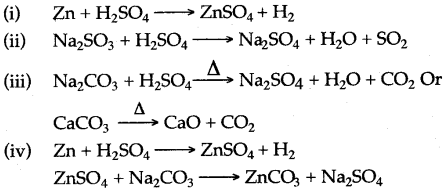Question 21: Which of the following salts give acidic solutions, alkaline solutions, and neutral solutions in water:
potassium nitrate, KNO3; ammonium sulphate, (NH4)2SO4; potassium carbonate, K2CO3; sodium chloride, NaCl; sodium acetate, CH3COONa; and copper sulphate, CuSO4.
(i) Acidic solutions ………………………………………………..
(ii) Alkaline solutions …………………………………………….
(iii) Neutral solutions …………………………………………….
Answer: (i) Acidic solutions: ammonium sulphate; (NH4)2SO4, copper sulphate (CuSO4).
(ii) Alkaline solutions: potassium carbonate; (K2CO3), sodium acetate (CH3COONa).
(iii) Neutral solutions: potassium nitrate; (KNO3), sodium chloride (NaCl).

Question 22: (i) Name four soluble salts.
(ii) Name four insoluble salts.
Answer: (i) (a) Zinc sulphate. (b) Iron (II) sulphate.
(c) Copper (II) sulphate. (d) Sodium sulphate.
(ii) (a) Calcium carbonate. (b) Lead sulphate.
(c) Silver chloride. (d) Barium sulphate.

Question 23: (i) What happens to the crystals of washing soda when exposed to air? Name the phenomenon exhibited.
(ii) Classify the following compounds into: Deliquescent, Efflorescent, None of these (a) Magnesium chloride crystals, (b) Zinc chloride crystals,
(c) Lead nitrate crystals, (d) Ferrous sulphate crystals,
(e) Lead chloride, (f) Copper sulphate crystals,
(g) Zinc hydroxide, (h) Magnesium chloride.
Answer: (i) When exposed to air washing soda crystals lose their water of crystallization and become amorphous.
∴   The phenomenon is called efflorescence.
(ii) (a) Deliquescent (b) Deliquescent (c) None of these
(d) None of these (e) None of these (f) Efflorescent
(g) None of these (h) Highly deliquescent.

Question 24: Answer the questions given below, relating your answers only to salts given in the following list: Sodium chloride, calcium chloride, copper sulphate.5-water.
(i) What name is given to the water in compound copper sulphate. 5-water?
(ii) If copper sulphate. 5-water is heated, the water is driven off leaving anhydrous copper sulphate.
(a) What is the colour of anhydrous copper sulphate?
(b) By what means, other than heating, could you dehydrate copper sulphate. 5-water and obtain anhydrous copper sulphate?
(iii) What is deliquescence.
(iv) Which one of the salts in the given list is deliquescent?
(ii) (a) White. (b) By adding cone. H2SO4.
(iii) Compounds that take up enough water from the air to dissolve in the water, are called deliquescent, e.g. Calcium chloride (CaCl2) and sodium hydroxide are deliquescent.
(iv) Calcium chloride.

Question 25: CuSO4.5H20, Na2CO3.10H2O, CaO, and anhydrous calcium chloride are chemicals commonly available in the laboratory. Answer the following questions relating your answer to the list of chemicals given above.
(i) Which salt is blue in color?
(ii) Which salt is efflorescent in nature?
(iii) Which salt is hygroscopic in nature?
(iv) Which salt is deliquescent in nature.
(v) State your observations when a solution of calcium chloride is mixed with a solution of sodium carbonate.
Answer: (i) CuSO4. 5H2O is blue in colour.
(ii) Na2CO3.10H2O is efflorescent in nature.
(iii) CaO is hygroscopic in nature.
(iv) Anhydrous calcium chloride is deliquescent in nature.
(v) A white ppt. of calcium carbonate appears when the solutions of CaCl2 and Na2CO3 are mixed. The white ppt. gradually settles at the base of the test tube.

Question 26: Answer the following questions, relating your answers only to salts in the list given below :
Anhydrous calcium chloride, copper sulphate. 5H2O, sodium carbonate. 10-water.
(i) Which compound is efflorescent?
(ii) Which compound is blue in colour?
(iii) Which compound is deliquescent?
(iv) What would be seen on mixing a solution of calcium chloride with a solution of sodium carbonate?
(v) Write the balanced equation for the reaction occurring when a solution of calcium chloride is mixed with a solution of sodium carbonate.
(ii) Copper sulphate. 5H2O.
(iii) Anhydrous calcium chloride.
(iv) A white precipitate of calcium carbonate is seen.
(v) CaCl2(aq.) + Na2CO3 (aq.) —> CaCO3 (White ppt) + 2NaCl (aq.)

Question 27: (i) Give chemical names of the following:
(a) Green vitriol (b) Blue vitriol (c) White vitriol.
(ii) State the colour of the following salts:
(a) Copper chloride (b) Ferric chloride
(c) Copper nitrate (d) Lead nitrate
(e) Magnesium carbonate (f) Zinc hydroxide
(iii) State the colours of the aqueous solution of the following salts:
(a) Calcium sulphate crystals (b) Ferrous chloride crystals
(c) Ferric chloride crystals (d) Ferrous sulphate crystals
(e) Ferric sulphate crystals (f) Copper sulphate crystals.
Answer: (i) (a) Green vitriol—Ferrous sulphate,
(b) Blue vitriol—Copper sulphate
(c) White vitriol—Zinc sulphate.
(ii) (a) Brown (anhydrous) (b) Black (anhydrous) (c) Blue
(d) White (e) White (f) White
(iii) (a) Colourless (b) Blue green (c) Yellow
(d) Green (e) Yellowish white (f) Blue.

### Figure/Table Based Questions

Question 1: Draw the structure of the stable positive ion formed when an acid dissolves in water.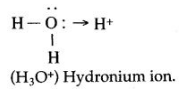Question 2: Some methods used for the laboratory preparation of salts are :
A : metal + acid
B : carbonate + acid
C : precipitation (double decomposition)
D : direct combination
E: titration
Copy and complete the following table:

 Salt Method of Preparation Ammonium sulphateCalcium carbonateIron (III) chlorideLead nitrateZinc sulphate

 Salt Method of Preparation Ammonium sulphateCalcium carbonateIron (III) chlorideLead nitrateZinc sulphate E: TitrationC: PrecipitationD: Direct CombinationB : Carbonate + acidA : Metal + acid

### Reasoning Based Questions

Question 1: Hydrochloric acid is considered a strong acid whereas acetic acid is a weak acid. Why?
Answer: Hydrochloric acid is considered a strong acid because it dissociates completely in water.Acetic acid is a weak acid as it-dissociates partially when dissolved in water. Most of its molecules remain in molecular form in the solution.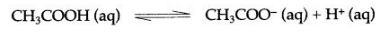Question 2: Why dilute sulphuric acid is a stronger acid than concentrated sulphuric acid?
Answer: The presence of water in dilute sulphuric acid increases the hydrogen ion concentration. Hence it is a stronger acid than concentrated sulphuric acid which contains comparatively less water.

Question 3: Acetic acid is monobasic. Why?
Answer: Acetic acid is monobasic because it has one dissociable hydrogen ion and combines with one hydroxyl ion of the base to give a single salt and water.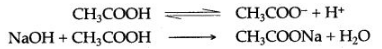Question 4: Carbonic acid is a dibasic acid. Why?
Answer: Carbonic acid (H2CO3) is a dibasic acid because it has two replaceable hydrogen atoms and hence it combines with two hydroxyl groups of the bases to form two kinds of salt and water.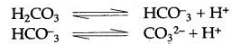The displacement of two hydrogen atoms takes place in two steps.

Question 5: Sodium hydroxide is a monoacidic base. Why?
Answer: Sodium hydroxide is a mono-acidic base as it combines with only one hydrogen ion.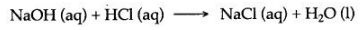Question 6: An aqueous solution of the salt ammonium chloride is acidic in nature while an aqueous solution of sodium chloride is neutral. Why?
Answer: Ammonium chloride is a salt of a weak base and strong acid, it undergoes salt hydrolysis to produce an acidic solution whereas sodium chloride is a salt of strong acid and strong base, it does not undergo salt hydrolysis hence its solution remains neutral.

Question 7: An aqueous solution of the zinc sulphate acidic in nature. Why?
Answer: When zinc sulphate is dissolved in water, it is hydrolysed to form sulphuric acid and zinc hydroxide. Sulphuric acid is a strong acid, while zinc hydroxide is a weak base, hence, the solution is acidic in nature.Question 8: An aqueous solution of ammonium acetate, neutral in nature. Why?
Answer: Ammonium acetate, when dissolved in water is hydrolysed to form ammonium hydroxide and acetic acid. Ammonium hydroxide and acetic acid both are weak alkali and acid respectively, hence the solution is neutral in nature.

Question 9: An aqueous solution of sodium carbonate is alkaline and that of ammonium chloride is acidic in behaviour. Why?
Answer: Both of these salts react with water. Sodium carbonate reacts with water, producing a strong alkali, sodium hydroxide and a weak acid, carbonic acid. Hence, the solution is alkaline: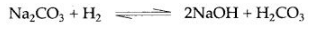Ammonium chloride reacts with water to produce ammonium hydroxide, weak alkali, and hydrochloric acid, a strong acid. Hence the solution is acidic: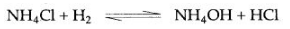Question 10: A universal indicator is more meaningful than an ordinary indicator.
Answer: A universal indicator is better than an ordinary indicator as it not only shows whether the solution is acidic or basic but also gives the pH values (approx.) by giving a wide range of colours corresponding to different pH values.

Question 11: The heat of neutralization of a strong acid with a strong base is always the same. Why?
Answer: Strong acids, strong bases, and their salts ionize completely in the solution. Consider the neutralization of hydrochloric acid by sodium hydroxide, which is as follows: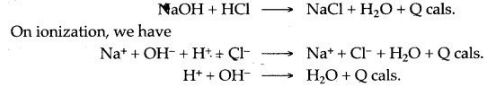This shows that the heat of neutralization of a strong acid by a strong base is nothing, but the heat of formation of a water molecule from hydrogen and hydroxyl ions, hence it is in the same and fixed quantity.

Question 12: Lime juice has a sour taste while lime water is slightly bitter. Why?
Answer: Lime juice contains citric acid which imparts a sour taste to it while lime water is alkaline and hence bitter to taste.

Question 13: While mixing strong sulphuric acid and water, why is the acid always poured slowly into the water instead of water into the acid?
Answer: When concentrated sulphuric acid is mixed with water, it evolves a considerable amount of heat. This heat is liberated due to the hydration of hydrogen ions derived from the acid.
H+ + H20  ⟶  H3O+ + Heat.
Due to the production of heat, the water may be vaporised into steam and spill out corrosive drops of acid. To avoid this, the acid is always slowly added to water.

Question 14: It is necessary to find out the ratio of reactants required in the preparation of sodium sulphate. Why?
Answer: In the preparation of sodium sulphate, sodium hydroxide and sulphuric acid are both soluble and excess of any of them can not be removed by filtration. Thus it is necessary to find out the ratio of the solutons of the two reactants required for complete neutralisations.

Question 15: Lead chloride can not be prepared by the action of hydrochloric acid on lead sulphate directly. Why?
Answer: An insoluble salt can not be prepared from another insoluble salt and since both lead chloride and lead sulphate are insoluble salt, we can not prepare lead chloride by the action of hydrochloric acid on lead sulphate directly.

Question 16: Sodium sulphite (Na2SO3) and sodium hydrogen sulphite (NaHSO3) are salts of sodium but sodium sulphite is called a normal salt and sodium hydrogen sulphite is called an acid salt. Why?
Answer: Sodium hydrogen sulphite has replaceable hydrogen in its molecule so it is called an acid salt but sodium sulphite does not have, so it is called a normal salt.Question 17: Fused calcium chloride used in a desiccator. Why?
Answer: Calcium chloride is a deliquescent substance, it absorbs water up to such an extent that it finally dissolves in it and moreover, it does not react with water. When calcium chloride is put in a desiccator, it absorbs all water vapours present in it and thus dry atmosphere is created inside.

Question 18: Why zinc chloride is stored in air-tight bottles.
Answer: Zinc chloride absorbs moisture from the atmosphere and turns into a solution because it is a deliquescent substance. In order to prevent this, it is stored in air-tight bottles.

Question 19: The solution of sodium carbonate is alkaline in nature. Why?
Answer: Sodium carbonate when dissolved in water is hydrolysed to form a weak acid, carbonic acid, and a strong base sodium hydroxide. Hence, an aqueous solution of sodium carbonate is alkaline in nature.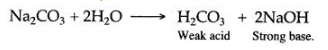Question 20: Why fused calcium chloride is used in the preparation of FeCl3?
Answer: FeCl3 is highly deliquescent, so it is kept dry with the help of fused calcium chloride.

### Chemical Tests

Question: How will you distinguish between the following pairs of compounds:
1. Iron (II) chloride and Iron (III) chloride.
2. Lead nitrate and copper nitrate.
3. Zinc oxide and calcium oxide.
4. Sodium carbonate and sodium nitrate.
5. Sodium sulphate and sodium sulphite.
1. Iron (II) chloride is dissolved in water and then sodium hydroxide is added. A dirty green precipitate is obtained which confirms the presence of iron (II) chloride.Iron (IK) chloride is also dissolved in water and then sodium hydroxide solution is added. A reddish brown precipitate is obtained, which confirms the presence of iron (HI) chloride.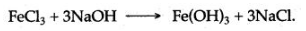2.
Lead nitrate is dissolved in water and then sodium hydroxide solution is added, a white precipitate is obtained, which is soluble in excess of sodium hydroxide.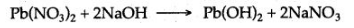Copper nitrate is dissolved in water and sodium hydroxide solution is added, a light blue precipitate of copper hydroxide is obtained.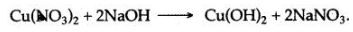3.
Zinc oxide is dissolved in dilute hydrochloric acid to form zinc chloride and then sodium hydroxide solution is added, a white precipitate is obtained, which is soluble in excess of sodium hydroxide to form a clear solution.Calcium chloride is obtained by dissolving calcium oxide in dilute hydrochloric acid. To the solution of calcium chloride, sodium hydroxide solution is added, a white precipitate of calcium hydroxide is obtained, which is insoluble even in the excess of sodium hydroxide.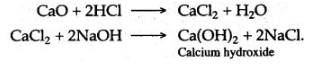4. Sodium carbonate when treated with dilute hydrochloric acid, a vigorous reaction takes place and a colourless, odourless gas carbon dioxide is evolved which turns lime water milky.Sodium nitrate when mixed with copper filings and heated with concentrated sulphuric acid, reddish-brown fumes of nitrogen dioxide are evolved.5.
Sodium sulphate is dissolved in water and barium chloride solution is added, an insoluble white precipitate of barium sulphate is obtained.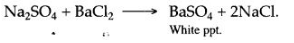Sodium sulphite is warmed with dilute sulphuric acid, a colourless gas with a pungent and suffocating smell is evolved. When this gas is bubbled through an acidified solution of potassium dichromate, it turns its colour from orange to green.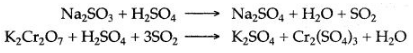### Balancing/Writing the Chemical Equations

Question 1: Write correctly balanced equations for the following reactions :
1. Molten sodium and chlorine.
2. Nitrogen and oxygen, when lightning strikes.
3. Iron and dilute sulphuric acid.
4. Decomposition of hypochlorous acid in sunlight.
5. Decomposition of potassium nitrate.
6. Sodiumlfubsulphate is reacted with dilute hydrochloric acid.
7. Calcium bicarbonate reacts with dilute hydrochloric acid.
8. Dilute sulphuric acid is poured over sodium sulphate
10. Zinc is heated with sodium hydroxide solution.
12. Copper sulphate from copper and concentrated sulphuric acid.
14. Ammonium sulphate from ammonia and dilute sulphuric acid.
15. Sodium chloride from sodium carbonate solution and dilute hydrochloric acid.
16. Magnesium and dilute sulphuric acid.
17. Zinc carbonate and dilute sulphuric acid.
18. Copper oxide and dilute sulphuric acid.
19. Ferric hydroxide reacts with nitric acid.
20. Zinc oxide dissolves in sodium hydroxide.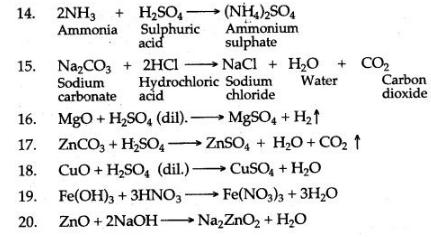Question 2: Write the equation for the laboratory preparation of the following salts
1. Iron (II) sulphate from iron.
2. Copper sulphate from copper.
4. Sodium sulphate from sodium carbonate.
5. Copper sulphate from copper (II) oxide.
6. Iron (in) chloride from iron.
7. Potassium sulphate from potassium hydroxide solution.
9. Zinc sulphate from zinc.
10. Sodium sulphate from sodium hydroxide.
12. Copper (II) sulphate from copper carbonate.
13. Calcium carbonate from calcium chloride.
14. Sodium sulphate from sodium carbonate
15. Zinc carbonate from zinc nitrate.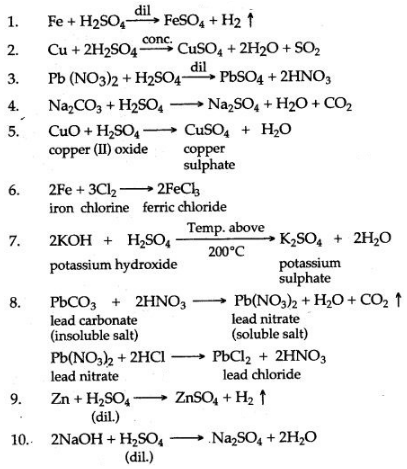Question 3: Study the following conversion schemes:
1. Give the equations for the following conversions A to E.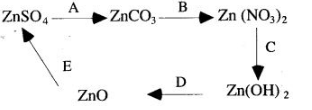2.
Refer to the flow chart diagram below and give balanced equations with conditions, if any, for the following conversions A to D.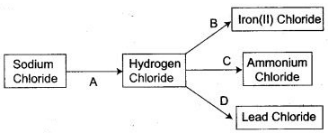3.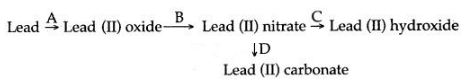(i) For each of the conversions A to D in the above state briefly how the conversions can be carried out.
(ii) Write equations for the conversions.
4. For each of the conversions in the scheme given below, state briefly in words or by means of a chemical equation, how the conversion is carried out?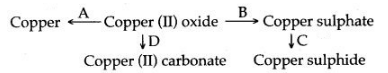5.
How are the following conversions carried out ? Give balanced chemical equations only.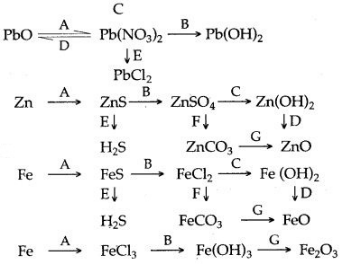3. [A] Lead (II) oxide, when heated with powdered coke, reduced to metallic lead and carbon monoxide is formed.
PbO+C  ⟶   Pb+CO
[B] Lead (II) oxide, when dissolved in the dilute nitric acid, lead nitrate is formed.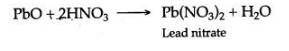[C] Lead (U) nitrate is dissolved in water and then sodium hydroxide solution is added, a white precipitate of lead hydroxide is obtained.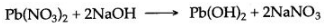[D] Lead (II) nitrate is dissolved in water and then concentrated soLution of sodium carbonate is added, a white precipitate of lead (Il) carbonate is obtained.4.
[A] Copper (II) oxide, when heated with coke powder is reduced to metallic copper.[B] Copper (II) oxide is dissolved in dilute sulphuric acid to form copper sulphate.[C] Copper sulphate is dissolved in water and hydrogen sulphide gas is passed, a black precipitate of copper sulphide is formed.[D] Copper (II) oxide is dissolved in dilute hydrochloric acid to form copper chloride. To the solution of copper chloride, when a saturated solution of sodium carbonate is added, a light blue precipitate of copper (II) carbonate is formed.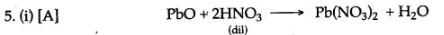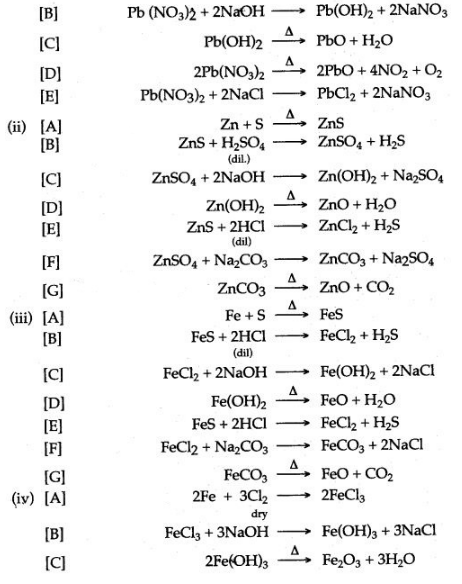Question 4: How can the following be converted to the respective chloride, sulphate, and nitrate salts? Give equations.
1. Magnesium, 2. Zinc, 3. Iron, 4. Lead carbonate, 5. Copper oxide, 6. Potassium hydroxide, 7. Copper hydroxide, 8. Ammonium hydroxide.